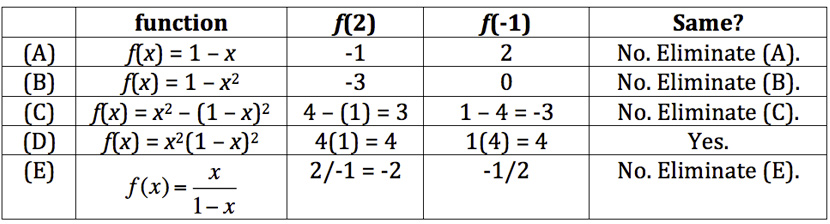# GMAT Problem Solving Strategy: Test Cases

by , Mar 17, 2015If youre going to do a great job on the GMAT, then youve got to know how to Test Cases. This strategy will help you on countless quant problems.

This technique is especially useful for Data Sufficiency problems, but you can also use it on some Problem Solving problems, like the GMATPrep problem below. Give yourself about 2 minutes. Go!

* For which of the following functions f is [pmath]f(x)=f(1-x)[/pmath] for all x?

(A) [pmath]f(x)=1-x[/pmath]

(B) [pmath]f(x)=1-x^2[/pmath]

(C) [pmath]f(x)=x^2-(1-x)^2[/pmath]

(D) [pmath]f(x)=x^2(1-x)^2[/pmath]

(E) [pmath]f(x)=x/{1-x}[/pmath]

Testing Cases is mostly what it sounds like: you will test various possible scenarios in order to narrow down the answer choices until you get to the one right answer. Whats the common characteristic that signals you can use this technique on problem solving?

The most common language will be something like Which of the following must be true? (or could be true)

The above problem doesnt have that language, but it does have a variation:you need to find the answer choice for which the given equation is true for all x, which is the equivalent of asking for which answer choice the given equation is always, or must be, true.

All right, so how are we actually going to test this thing? Here are the steps:

First, choose numbers to test in the problem.

Second, double check that you have selected a valid case. If the problem provided any restrictions, make sure that you didnt pick numbers that violate those restrictions.

But wait, Im not even sure I understand the question yet. Lets take a minute to wrap our heads around the function notation. Whats the significance of saying that [pmath]f(x) = f(1-x)[/pmath]?

The f letter signals a function. Normally, youd see something like this:

[pmath]f(x) = 3x + 19[/pmath]

What thats saying is every time I give you a specific value for x, multiply it by 3 and then add 19.

The question stem, though, has something weird: its got that [pmath]f(x)[/pmath] thing on both sides of the equation. Whats that all about?

Glance down at the answers. Theyre all normal functions (that is, they look the way we expect functions to look). So theres really only one[pmath]f(1-x)[/pmath]function for each answer, but were supposed to solve the function in two different ways. First, we solve the function for [pmath]f(x)[/pmath]. Then, we solve the same function for [pmath]f(1-x)[/pmath]. If those two solutions match, then the answer choice stays in. If the two solutions do not match, then we get to cross that answer choice off.

All right, ready to try the first case? Pick something easy for x, making sure you follow any restrictions given by the problem, and test those answer choices.

Lets try [pmath]x = 2[/pmath] first.

Case #1:

[pmath]x = 2[/pmath]

[pmath](1 -x) = -1[/pmath]

The question is: [pmath]f(x) = f(1-x)[/pmath]?

Rewrite it: does [pmath]f(2) = f(-1)[/pmath]?Lucky! In this case, we had to try only one number to get rid of the 4 wrong cases. More typically, youll try 2 or sometimes 3 cases in order to get down to a single answer.